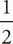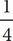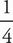# SAT Math Multiple Choice Question 164: Answer and Explanation

### Test Information

Question: 164

14. If r = (a + b)2 and s = -4ab + 3b, what is r - 2s in terms of a and b ?

• A.a2 + b2 - 7ab - 6b
• B.a2 + b2 - 7ab + 6b
• C.a2 + b2 + 9ab - 6b
• D.a2 + b2 + 9ab - 6b

C Whenever there are variables in the question and in the answers, think Plugging In. If a = 2 and b = 3, r = [(2) + 3]2 = (1 + 3)2 = 16, and s = -4(2)(3) + 3(3) = -24 + 9 = -15. The expression r - 2s becomes 16 - 2(-15) = 16 + 30 = 46. Plug 2 in for a and 3 in for b in each of the answers to see which answer equals the target number of 46. Choice (A) becomes(22) + 32 - 7(2)(3) - 6(3) = 1 + 9 - 42 - 18 = -50. This does not match the target number, so eliminate (A). Choice (B) becomes(22) + 32 - 7(2)(3) + 6(3) = 1 + 9 - 42 + 18 = -14. Eliminate (B). Choice (C) becomes(22) + 32 + 9(2)(3) - 6(3) = 1 + 9 + 54 - 18 = 46. Keep (C), but check (D) just in case it also works. Choice (D) is the same as (C) except for the coefficient on the a2 term, so it can't equal 46. Eliminate (D). The correct answer is (C).